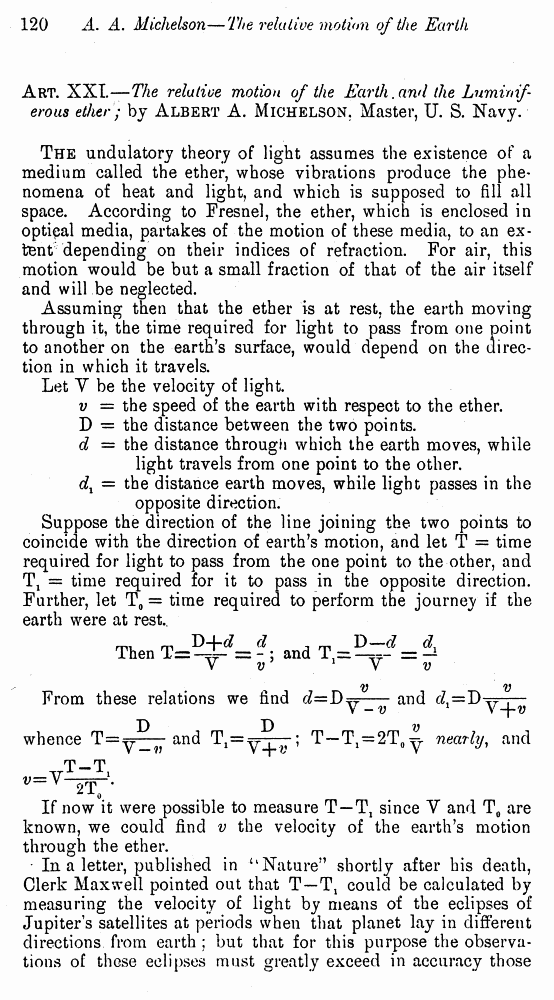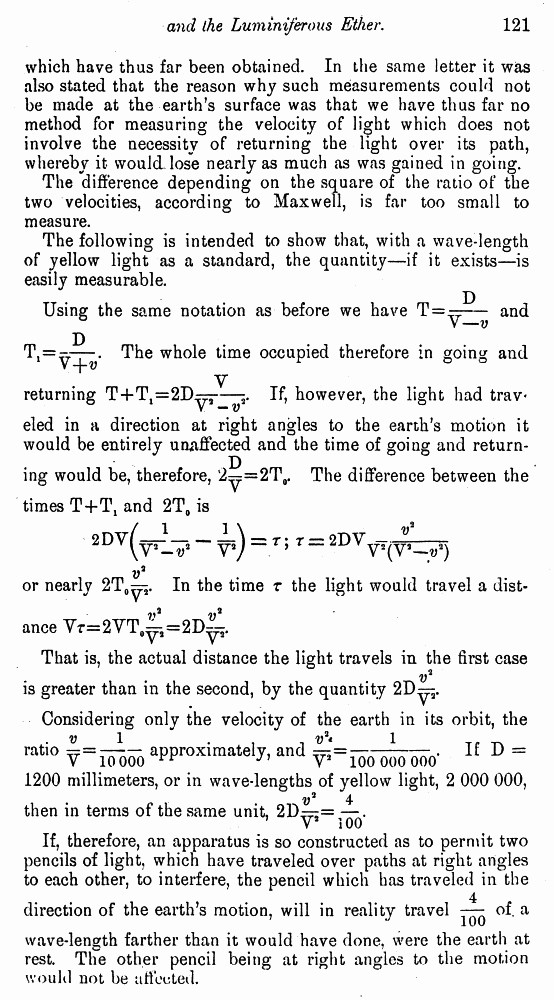# Albert A. Michelson. The Relative Motion of the Earth and the Luminiferous Ether // American Journal of Science, 1881, 22: Page 120

В начало   Другие форматы   <<<     Страница 1   >>>1 2  3  4  5  6  7  8  9  10 Art. XXI.—The relative motion of the Earth, and the Luminiferous ether; by Albert A. Michelson, Master, U. S. Navy. The undulatory theory of light assumes the existence of a medium called the ether, whose vibrations produce the phenomena of heat and light, and which is supposed to fill all space. According to Fresnel, the ether, which is enclosed in optical media, partakes of the motion of these media, to an extent depending on their indices of refraction. For air, this motion would be but a small fraction of that of the air itself and will be neglected. Assuming then that the ether is at rest, the earth moving through it, the time required for light to pass from one point to another on the earth’s surface, would depend on the direction in which it travels. Let Y be the velocity of light. v = the speed of the earth with respect to the ether. D = the distance between the two points. d — the distance through which the earth moves, while light travels from one point to the other. d1 = the distance earth moves, while light passes in the opposite direction. Suppose the direction of the line joining the two points to coincide with the direction of earth’s motion, and let T = time required for light to pass from the one point to the other, and T1 = time required for it to pass in the opposite direction. Further, let T0 = time required to perform the journey if the earth were at rest.. Thenand From these relations we findand whenceandnearly, and If now it were possible to measure T — T1 since V and T0 are known, we could find v the velocity of the earth’s motion through the ether. In a letter, published in “Nature” shortly after his death, Clerk Maxwell pointed out that T —T1 could be calculated by measuring the velocity of light by means of the eclipses of Jupiter’s satellites at periods when that planet lay in different directions from earth ; but that for this purpose the observations of these eclipses must greatly exceed in accuracy those which have thus far been obtained. In the same letter it was also stated that the reason why such measurements could not be made at the earth’s surface was that we have thus far no method for measuring the velocity of light which does not involve the necessity of returning the light over its path, whereby it would lose nearly as much as was gained in going. The difference depending on the square of the ratio of the two velocities, according to Maxwell, is far too small to measure. The following is intended to show that, with a wave-length of yellow light as a standard, the quantity—if it exists—is easily measurable. Using the same notation as before we haveand The whole time occupied therefore in going and returningIf, however, the light had trav eled in a direction at right angles to the earth’s motion it would be entirely unaffectedand the time of going and returning would be, therefore,The difference between the times T+T1 and 2T0 is or nearlyIn the timethe light would travel a dist ance That is, the actual distance the light travels in the first case is greater than in the second, by the quantity Considering only the velocity of the earth in its orbit, the ratioapproximately, andIf D = 1200 millimeters, or in wave-lengths of yellow light, 2 000 000, then in terms of the same unit, If, therefore, an apparatus is so constructed as to permit two pencils of light, which have traveled over paths at right angles to each other, to interfere, the pencil which has traveled in the direction of the earth’s motion, will in reality travelof. a wave-length farther than it would have done, were the earth at rest. The other pencil being at right angles to the motion would not be affected.